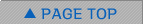Events / Media

## Data Assimilation Seminar Series

### Prof. Hiromichi Nagao (Feb. 7, 2017, 16:00-)

Affiliation Earthquake Research Institute, The University of Tokyo / Department of Mathematical Informatics, Graduate School of Information Science and Technology, The University of Tokyo Data assimilation for massive autonomous systems based on a second-order adjoint method We propose an adjoint-based data assimilation method for massive autonomous models that produces optimum estimates and their uncertainties within reasonable computation time and resource constraints. The uncertainties are given as several diagonal elements of an inverse Hessian matrix, which is the covariance matrix of a normal distribution that approximates the target posterior probability density function in the neighborhood of the optimum. Conventional algorithms for deriving the inverse Hessian matrix require O(CN2+N3) computations and O(N2) memory, where N is the number of degrees of freedom of a given autonomous system and C is the number of computations needed to simulate time series of suitable length. The proposed method using a second-order adjoint method allows us to directly evaluate the diagonal elements of the inverse Hessian matrix without computing all of its elements. This drastically reduces the number of computations to O(C) and the amount of memory to O(N) for each diagonal element. The proposed method is validated through numerical tests using a massive two-dimensional Kobayashi phase-field model. We confirm that the proposed method correctly reproduces the parameter and initial state assumed in advance, and successfully evaluates the uncertainty of the parameter. Link to PDF file